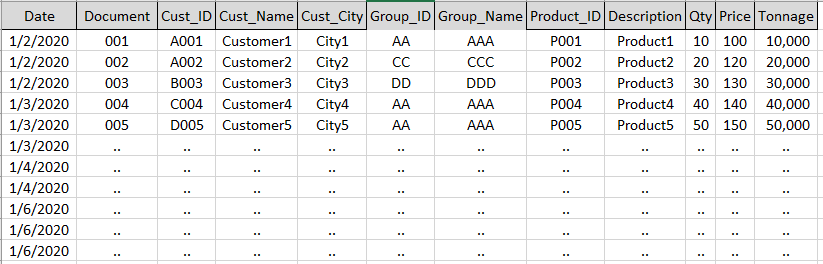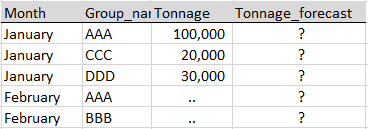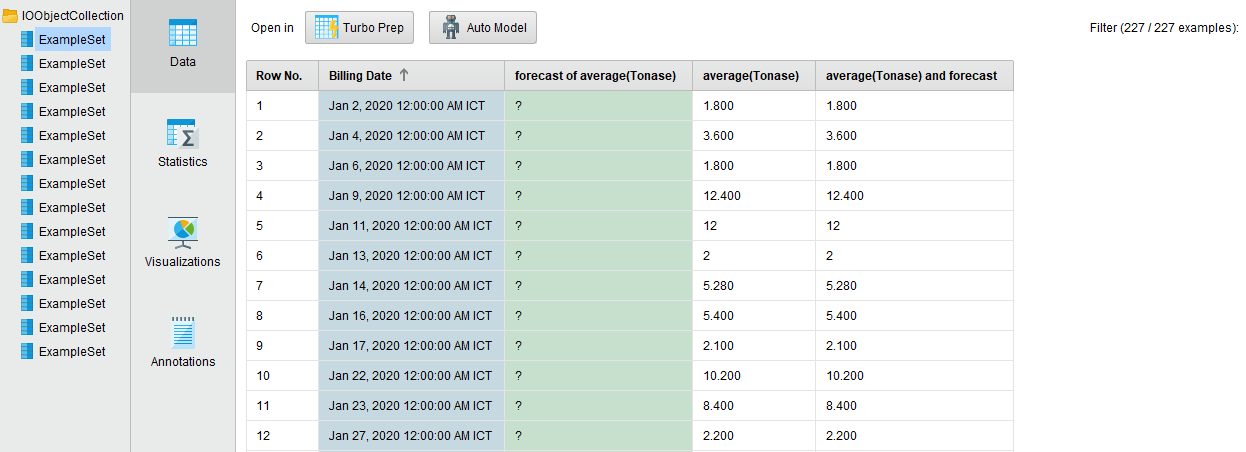# Sales Forecasting using ARIMA

Member Posts: 2Learner I
in Help
Hi everyone,

I'm still new to using RapidMiner and having problems when trying to deploy a sales forecast model using ARIMA. My data is a one-year sales transaction (60,000+ records).Label: Tonnage
The purpose is to forecast tonnage but need to know the product group (Group_Name) column

For example, the forecast should be like this (assume - maybe need an additional column for more information)I try to follow Dr. Fabian Temme's video about time series forecasting but still no luck.

Best regards,
ScottBett
Tagged:

• Moderator, RapidMiner Certified Analyst, Member, University Professor Posts: 555Unicorn
Hello, @ScottBett8

Sorry nobody has chimed in. Do you still have issues with ARIMA forecasting? I might be able to help.

All the best,

Rod.
• Administrator, Employee, RapidMiner Certified Analyst, Member Posts: 214Unicorn
Hi @ScottBett8,

You could use a Group Into Collection and a Loop Collection operator for your use case.
It seems that you need to forecast the Qty on your problems since the Tonage is the result of Qty (variable a predictable) and Price (you may already have those prices or may need to predict ahead of time)

You'll need to install the operator toolbox extension to get access to the Group Into Collection operator.

I would also suggest you try the Forecasting Extension.
https://community.rapidminer.com/discussion/comment/66543#Comment_66543

```<?xml version="1.0" encoding="UTF-8"?><process version="9.9.002">
<context>
<input/>
<output/>
<macros/>
</context>
<operator activated="true" class="process" compatibility="9.9.002" expanded="true" name="Process">
<parameter key="logverbosity" value="init"/>
<parameter key="random_seed" value="-1"/>
<parameter key="send_mail" value="never"/>
<parameter key="process_duration_for_mail" value="30"/>
<parameter key="encoding" value="SYSTEM"/>
<process expanded="true">
<operator activated="true" class="subprocess" compatibility="9.9.002" expanded="true" height="82" name="Fake_Data" width="90" x="112" y="34">
<process expanded="true">
<operator activated="true" class="utility:create_exampleset" compatibility="9.9.002" expanded="true" height="68" name="Customers" width="90" x="45" y="34">
<parameter key="generator_type" value="comma separated text"/>
<parameter key="number_of_examples" value="100"/>
<parameter key="use_stepsize" value="false"/>
<list key="function_descriptions"/>
<list key="numeric_series_configuration"/>
<list key="date_series_configuration"/>
<list key="date_series_configuration (interval)"/>
<parameter key="date_format" value="yyyy-MM-dd HH:mm:ss"/>
<parameter key="time_zone" value="SYSTEM"/>
<parameter key="input_csv_text" value="Customer&#10;Customer_A&#10;Customer_B&#10;Customer_C&#10;Customer_D&#10;Customer_E&#10;Customer_F"/>
<parameter key="column_separator" value=","/>
<parameter key="parse_all_as_nominal" value="false"/>
<parameter key="decimal_point_character" value="."/>
<parameter key="trim_attribute_names" value="true"/>
</operator>
<operator activated="true" class="loop_examples" compatibility="9.9.002" expanded="true" height="103" name="Loop Examples" width="90" x="179" y="34">
<parameter key="iteration_macro" value="example"/>
<process expanded="true">
<operator activated="true" class="extract_macro" compatibility="9.9.002" expanded="true" height="68" name="Extract Macro" width="90" x="45" y="34">
<parameter key="macro" value="customer"/>
<parameter key="macro_type" value="data_value"/>
<parameter key="statistics" value="average"/>
<parameter key="attribute_name" value="Customer"/>
<parameter key="example_index" value="%{example}"/>
</operator>
<operator activated="true" class="utility:create_exampleset" compatibility="9.9.002" expanded="true" height="68" name="Days" width="90" x="179" y="34">
<parameter key="generator_type" value="date series"/>
<parameter key="number_of_examples" value="365"/>
<parameter key="use_stepsize" value="false"/>
<list key="function_descriptions"/>
<list key="numeric_series_configuration"/>
<list key="date_series_configuration">
<parameter key="Date" value="2020-01-01.2020-12-31"/>
</list>
<list key="date_series_configuration (interval)"/>
<parameter key="date_format" value="yyyy-MM-dd"/>
<parameter key="time_zone" value="SYSTEM"/>
<parameter key="column_separator" value=","/>
<parameter key="parse_all_as_nominal" value="false"/>
<parameter key="decimal_point_character" value="."/>
<parameter key="trim_attribute_names" value="true"/>
</operator>
<operator activated="true" class="generate_data" compatibility="9.9.002" expanded="true" height="68" name="Sales" width="90" x="179" y="136">
<parameter key="target_function" value="random"/>
<parameter key="number_examples" value="365"/>
<parameter key="number_of_attributes" value="1"/>
<parameter key="attributes_lower_bound" value="20.0"/>
<parameter key="attributes_upper_bound" value="150.0"/>
<parameter key="gaussian_standard_deviation" value="10.0"/>
<parameter key="use_local_random_seed" value="false"/>
<parameter key="local_random_seed" value="1992"/>
<parameter key="datamanagement" value="double_array"/>
<parameter key="data_management" value="auto"/>
</operator>
<operator activated="true" class="select_attributes" compatibility="9.9.002" expanded="true" height="82" name="Select Attributes (3)" width="90" x="313" y="136">
<parameter key="attribute_filter_type" value="single"/>
<parameter key="attribute" value="label"/>
<parameter key="attributes" value=""/>
<parameter key="use_except_expression" value="false"/>
<parameter key="value_type" value="attribute_value"/>
<parameter key="use_value_type_exception" value="false"/>
<parameter key="except_value_type" value="time"/>
<parameter key="block_type" value="attribute_block"/>
<parameter key="use_block_type_exception" value="false"/>
<parameter key="except_block_type" value="value_matrix_row_start"/>
<parameter key="invert_selection" value="true"/>
<parameter key="include_special_attributes" value="true"/>
</operator>
<operator activated="true" class="blending:rename" compatibility="9.9.002" expanded="true" height="82" name="Rename" width="90" x="447" y="136">
<list key="rename attributes">
<parameter key="att1" value="Qty"/>
</list>
<parameter key="from_attribute" value=""/>
<parameter key="to_attribute" value=""/>
</operator>
<operator activated="true" class="operator_toolbox:merge" compatibility="2.11.000" expanded="true" height="103" name="Merge Attributes (2)" width="90" x="648" y="85">
<parameter key="handling_of_duplicate_attributes" value="rename"/>
<parameter key="handling_of_special_attributes" value="keep_first_special_other_regular"/>
<parameter key="handling_of_duplicate_annotations" value="rename"/>
</operator>
<operator activated="true" class="generate_attributes" compatibility="9.9.002" expanded="true" height="82" name="Generate Attributes (5)" width="90" x="782" y="85">
<list key="function_descriptions">
<parameter key="Customer" value="%{customer}"/>
<parameter key="Qty" value="round(Qty,0)"/>
</list>
<parameter key="keep_all" value="true"/>
</operator>
<connect from_port="example set" to_op="Extract Macro" to_port="example set"/>
<connect from_op="Days" from_port="output" to_op="Merge Attributes (2)" to_port="example set 1"/>
<connect from_op="Sales" from_port="output" to_op="Select Attributes (3)" to_port="example set input"/>
<connect from_op="Select Attributes (3)" from_port="example set output" to_op="Rename" to_port="example set input"/>
<connect from_op="Rename" from_port="example set output" to_op="Merge Attributes (2)" to_port="example set 2"/>
<connect from_op="Merge Attributes (2)" from_port="merged set" to_op="Generate Attributes (5)" to_port="example set input"/>
<connect from_op="Generate Attributes (5)" from_port="example set output" to_port="output 1"/>
<portSpacing port="source_example set" spacing="0"/>
<portSpacing port="sink_example set" spacing="0"/>
<portSpacing port="sink_output 1" spacing="0"/>
<portSpacing port="sink_output 2" spacing="0"/>
</process>
</operator>
<operator activated="true" class="append" compatibility="9.9.002" expanded="true" height="82" name="Append" width="90" x="313" y="34">
<parameter key="datamanagement" value="double_array"/>
<parameter key="data_management" value="auto"/>
<parameter key="merge_type" value="all"/>
</operator>
<operator activated="true" class="blending:sort" compatibility="9.9.002" expanded="true" height="82" name="Sort" width="90" x="447" y="34">
<list key="sort_by">
<parameter key="Date" value="ascending"/>
<parameter key="Customer" value="ascending"/>
</list>
</operator>
<connect from_op="Customers" from_port="output" to_op="Loop Examples" to_port="example set"/>
<connect from_op="Loop Examples" from_port="output 1" to_op="Append" to_port="example set 1"/>
<connect from_op="Append" from_port="merged set" to_op="Sort" to_port="example set input"/>
<connect from_op="Sort" from_port="example set output" to_port="out 1"/>
<portSpacing port="source_in 1" spacing="0"/>
<portSpacing port="sink_out 1" spacing="0"/>
<portSpacing port="sink_out 2" spacing="0"/>
</process>
</operator>
<operator activated="true" class="operator_toolbox:group_into_collection" compatibility="2.11.000" expanded="true" height="82" name="Group Into Collection" width="90" x="313" y="34">
<parameter key="group_by_attribute" value="Customer"/>
<parameter key="group_by_attribute (numerical)" value=""/>
<parameter key="sorting_order" value="alphabetical"/>
</operator>
<operator activated="true" class="loop_collection" compatibility="9.9.002" expanded="true" height="103" name="Loop Collection" width="90" x="514" y="34">
<parameter key="set_iteration_macro" value="false"/>
<parameter key="macro_name" value="iteration"/>
<parameter key="macro_start_value" value="1"/>
<parameter key="unfold" value="false"/>
<process expanded="true">
<operator activated="true" class="time_series:arima_trainer" compatibility="9.9.002" expanded="true" height="103" name="ARIMA" width="90" x="112" y="34">
<parameter key="time_series_attribute" value="Qty"/>
<parameter key="has_indices" value="true"/>
<parameter key="indices_attribute" value="Date"/>
<parameter key="p:_order_of_the_autoregressive_model" value="1"/>
<parameter key="d:_degree_of_differencing" value="0"/>
<parameter key="q:_order_of_the_moving-average_model" value="1"/>
<parameter key="estimate_constant" value="true"/>
<parameter key="main_criterion" value="aic"/>
</operator>
<operator activated="true" class="time_series:apply_forecast" compatibility="9.9.002" expanded="true" height="82" name="Apply Forecast" width="90" x="313" y="34">
<parameter key="forecast_horizon" value="12"/>
</operator>
<connect from_port="single" to_op="ARIMA" to_port="example set"/>
<connect from_op="ARIMA" from_port="forecast model" to_op="Apply Forecast" to_port="forecast model"/>
<connect from_op="Apply Forecast" from_port="example set" to_port="output 2"/>
<portSpacing port="source_single" spacing="0"/>
<portSpacing port="sink_output 1" spacing="0"/>
<portSpacing port="sink_output 2" spacing="0"/>
<portSpacing port="sink_output 3" spacing="0"/>
</process>
</operator>
<connect from_op="Fake_Data" from_port="out 1" to_op="Group Into Collection" to_port="exa"/>
<connect from_op="Group Into Collection" from_port="col" to_op="Loop Collection" to_port="collection"/>
<connect from_op="Loop Collection" from_port="output 1" to_port="result 1"/>
<portSpacing port="source_input 1" spacing="0"/>
<portSpacing port="sink_result 1" spacing="0"/>
<portSpacing port="sink_result 2" spacing="0"/>
</process>
</operator>
</process>```

• Member Posts: 2Learner I
edited July 19

Sorry for the late reply. Got caught up in my work for weeks.
I tried your suggestion. It was a good idea to try your approach. Qty unit of measure is in Kg (kilograms) and Tonase is converted to Tons. After I apply your XML, indeed 14 product groups are generated. But the forecast is empty.I also attach the XML
<?xml version="1.0" encoding="UTF-8"?><process version="9.9.002">
<context>
<input/>
<output/>
<macros/>
</context>
<operator activated="true" class="process" compatibility="9.9.002" expanded="true" name="Process">
<parameter key="logverbosity" value="init"/>
<parameter key="random_seed" value="-1"/>
<parameter key="send_mail" value="never"/>
<parameter key="process_duration_for_mail" value="30"/>
<parameter key="encoding" value="SYSTEM"/>
<process expanded="true">
<operator activated="true" class="retrieve" compatibility="9.9.002" expanded="true" height="68" name="Retrieve Data_2020" width="90" x="45" y="34">
<parameter key="repository_entry" value="Data_2020"/>
</operator>
<operator activated="true" class="select_attributes" compatibility="9.9.002" expanded="true" height="82" name="Select Attributes" width="90" x="179" y="34">
<parameter key="attribute_filter_type" value="subset"/>
<parameter key="attribute" value=""/>
<parameter key="use_except_expression" value="false"/>
<parameter key="value_type" value="attribute_value"/>
<parameter key="use_value_type_exception" value="false"/>
<parameter key="except_value_type" value="time"/>
<parameter key="block_type" value="attribute_block"/>
<parameter key="use_block_type_exception" value="false"/>
<parameter key="except_block_type" value="value_matrix_row_start"/>
<parameter key="invert_selection" value="false"/>
<parameter key="include_special_attributes" value="false"/>
</operator>
<operator activated="true" class="blending:sort" compatibility="9.9.002" expanded="true" height="82" name="Sort" width="90" x="313" y="34">
<list key="sort_by">
<parameter key="Billing Date" value="ascending"/>
</list>
</operator>
<operator activated="true" class="aggregate" compatibility="9.9.002" expanded="true" height="82" name="Aggregate" width="90" x="447" y="34">
<parameter key="use_default_aggregation" value="false"/>
<parameter key="attribute_filter_type" value="all"/>
<parameter key="attribute" value=""/>
<parameter key="attributes" value=""/>
<parameter key="use_except_expression" value="false"/>
<parameter key="value_type" value="attribute_value"/>
<parameter key="use_value_type_exception" value="false"/>
<parameter key="except_value_type" value="time"/>
<parameter key="block_type" value="attribute_block"/>
<parameter key="use_block_type_exception" value="false"/>
<parameter key="except_block_type" value="value_matrix_row_start"/>
<parameter key="invert_selection" value="false"/>
<parameter key="include_special_attributes" value="false"/>
<parameter key="default_aggregation_function" value="average"/>
<list key="aggregation_attributes">
<parameter key="Tonase" value="average"/>
</list>
<parameter key="count_all_combinations" value="false"/>
<parameter key="only_distinct" value="false"/>
<parameter key="ignore_missings" value="true"/>
</operator>
<operator activated="true" class="operator_toolbox:group_into_collection" compatibility="2.11.000" expanded="true" height="82" name="Group Into Collection" width="90" x="581" y="34">
<parameter key="group_by_attribute (numerical)" value=""/>
<parameter key="sorting_order" value="alphabetical"/>
</operator>
<operator activated="true" class="loop_collection" compatibility="9.9.002" expanded="true" height="82" name="Loop Collection" width="90" x="715" y="34">
<parameter key="set_iteration_macro" value="false"/>
<parameter key="macro_name" value="iteration"/>
<parameter key="macro_start_value" value="1"/>
<parameter key="unfold" value="false"/>
<process expanded="true">
<operator activated="true" class="time_series:arima_trainer" compatibility="9.9.002" expanded="true" height="103" name="ARIMA" width="90" x="112" y="34">
<parameter key="time_series_attribute" value="average(Tonase)"/>
<parameter key="has_indices" value="true"/>
<parameter key="indices_attribute" value="Billing Date"/>
<parameter key="p:_order_of_the_autoregressive_model" value="1"/>
<parameter key="d:_degree_of_differencing" value="0"/>
<parameter key="q:_order_of_the_moving-average_model" value="1"/>
<parameter key="estimate_constant" value="true"/>
<parameter key="main_criterion" value="aic"/>
</operator>
<operator activated="true" class="time_series:apply_forecast" compatibility="9.9.002" expanded="true" height="82" name="Apply Forecast" width="90" x="313" y="34">
<parameter key="forecast_horizon" value="12"/>
</operator>
<connect from_port="single" to_op="ARIMA" to_port="example set"/>
<connect from_op="ARIMA" from_port="forecast model" to_op="Apply Forecast" to_port="forecast model"/>
<connect from_op="Apply Forecast" from_port="example set" to_port="output 1"/>
<portSpacing port="source_single" spacing="0"/>
<portSpacing port="sink_output 1" spacing="0"/>
<portSpacing port="sink_output 2" spacing="0"/>
</process>
</operator>
<connect from_op="Retrieve Data_2020" from_port="output" to_op="Select Attributes" to_port="example set input"/>
<connect from_op="Select Attributes" from_port="example set output" to_op="Sort" to_port="example set input"/>
<connect from_op="Sort" from_port="example set output" to_op="Aggregate" to_port="example set input"/>
<connect from_op="Aggregate" from_port="example set output" to_op="Group Into Collection" to_port="exa"/>
<connect from_op="Group Into Collection" from_port="col" to_op="Loop Collection" to_port="collection"/>
<connect from_op="Loop Collection" from_port="output 1" to_port="result 1"/>
<portSpacing port="source_input 1" spacing="0"/>
<portSpacing port="sink_result 1" spacing="0"/>
<portSpacing port="sink_result 2" spacing="0"/>
</process>
</operator>
</process>

I tried using the Forecast Validation operator but cannot run.

Regards,
ScottBett8
• Moderator, RapidMiner Certified Analyst, Member Posts: 1,174Unicorn
Hi @ScottBett8,

Mmmh, it is expected that the first rows are not defined.
But can you confirm that  the entire column "forecast of average (Tonase)" contains only interrogation marks ?
Moreover can you share your dataset in order we can run the process, see what is going on and fix it ?

Regards,

Lionel